# Signal Generation and Visualization

This example shows how to generate widely used periodic and aperiodic waveforms, swept-frequency sinusoids, and pulse trains using functions available in Signal Processing Toolbox™.

### Periodic Waveforms

In addition to the `sin` and `cos` functions in MATLAB®, Signal Processing Toolbox™ offers other functions, such as `sawtooth` and `square`, that produce periodic signals.

The `sawtooth` function generates a sawtooth wave with peaks at $±1$ and a period of $2\pi$. An optional width parameter specifies a fractional multiple of $2\pi$ at which the signal's maximum occurs.

The `square` function generates a square wave with a period of $2\pi$. An optional parameter specifies duty cycle, the percent of the period for which the signal is positive.

Generate 1.5 seconds of a 50 Hz sawtooth wave with a sample rate of 10 kHz. Repeat the computation for a square wave.

```fs = 10000; t = 0:1/fs:1.5; x1 = sawtooth(2*pi*50*t); x2 = square(2*pi*50*t); subplot(2,1,1) plot(t,x1) axis([0 0.2 -1.2 1.2]) xlabel('Time (sec)') ylabel('Amplitude') title('Sawtooth Periodic Wave') subplot(2,1,2) plot(t,x2) axis([0 0.2 -1.2 1.2]) xlabel('Time (sec)') ylabel('Amplitude') title('Square Periodic Wave')```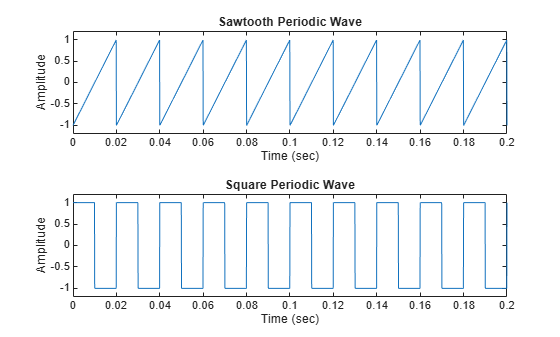### Aperiodic Waveforms

To generate triangular, rectangular and Gaussian pulses, the toolbox offers the `tripuls`, `rectpuls`, and `gauspuls` functions.

The `tripuls` function generates a sampled aperiodic, unit-height triangular pulse centered about t = 0 and with a default width of 1.

The `rectpuls` function generates a sampled aperiodic, unit-height rectangular pulse centered about t = 0 and with a default width of 1. The interval of nonzero amplitude is defined to be open on the right: `rectpuls(-0.5) = 1` while `rectpuls(0.5) = 0`.

Generate 2 seconds of a triangular pulse with a sample rate of 10 kHz and a width of 20 ms. Repeat the computation for a rectangular pulse.

```fs = 10000; t = -1:1/fs:1; x1 = tripuls(t,20e-3); x2 = rectpuls(t,20e-3); figure subplot(2,1,1) plot(t,x1) axis([-0.1 0.1 -0.2 1.2]) xlabel('Time (sec)') ylabel('Amplitude') title('Triangular Aperiodic Pulse') subplot(2,1,2) plot(t,x2) axis([-0.1 0.1 -0.2 1.2]) xlabel('Time (sec)') ylabel('Amplitude') title('Rectangular Aperiodic Pulse')```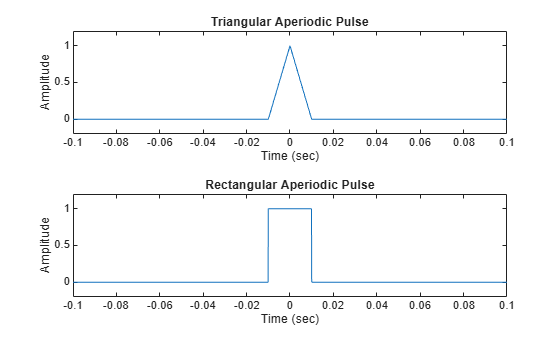The `gauspuls` function generates a Gaussian-modulated sinusoidal pulse with a specified time, center frequency, and fractional bandwidth.

The `sinc` function computes the mathematical sinc function for an input vector or matrix. The sinc function is the continuous inverse Fourier transform of a rectangular pulse of width $2\pi$ and unit height.

Generate a 50 kHz Gaussian RF pulse with 60% bandwidth, sampled at a rate of 1 MHz. Truncate the pulse where the envelope falls 40 dB below the peak.

```tc = gauspuls('cutoff',50e3,0.6,[],-40); t1 = -tc : 1e-6 : tc; y1 = gauspuls(t1,50e3,0.6);```

Generate the sinc function for a linearly spaced vector:

```t2 = linspace(-5,5); y2 = sinc(t2); figure subplot(2,1,1) plot(t1*1e3,y1) xlabel('Time (ms)') ylabel('Amplitude') title('Gaussian Pulse') subplot(2,1,2) plot(t2,y2) xlabel('Time (sec)') ylabel('Amplitude') title('Sinc Function')```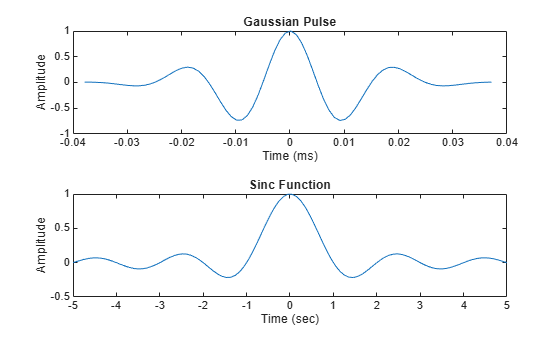### Swept-Frequency Waveforms

The toolbox also provides functions to generate swept-frequency waveforms such as the `chirp` function. Two optional parameters specify alternative sweep methods and initial phase in degrees. Below are several examples of using the `chirp` function to generate linear or quadratic, convex, and concave quadratic chirps.

Generate a linear chirp.

```t = 0:0.001:2; % 2 secs @ 1kHz sample rate ylin = chirp(t,0,1,150); % Start @ DC, cross 150Hz at t=1sec```

Generate a quadratic chirp.

```t = -2:0.001:2; % +/-2 secs @ 1kHz sample rate yq = chirp(t,100,1,200,'q'); % Start @ 100Hz, cross 200Hz at t=1sec```

Compute and display the spectrograms of the chirps.

```figure subplot(2,1,1) spectrogram(ylin,256,250,256,1E3,'yaxis') title('Linear Chirp') subplot(2,1,2) spectrogram(yq,128,120,128,1E3,'yaxis') title('Quadratic Chirp')```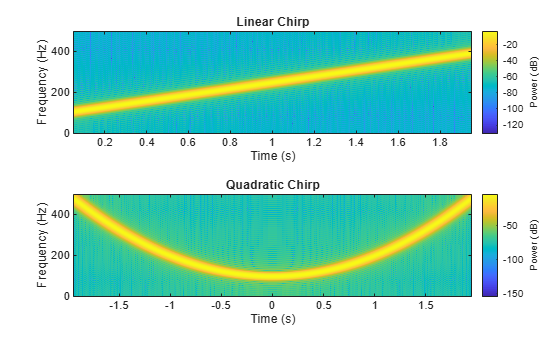Generate a convex quadratic chirp.

```t = -1:0.001:1; % +/-1 second @ 1kHz sample rate fo = 100; f1 = 400; % Start at 100Hz, go up to 400Hz ycx = chirp(t,fo,1,f1,'q',[],'convex');```

Generate a concave quadratic chirp.

```t = -1:0.001:1; % +/-1 second @ 1kHz sample rate fo = 400; f1 = 100; % Start at 400Hz, go down to 100Hz ycv = chirp(t,fo,1,f1,'q',[],'concave');```

Compute and display the spectrograms of the chirps.

```figure subplot(2,1,1) spectrogram(ycx,256,255,128,1000,'yaxis') title('Convex Chirp') subplot(2,1,2) spectrogram(ycv,256,255,128,1000,'yaxis') title('Concave Chirp')```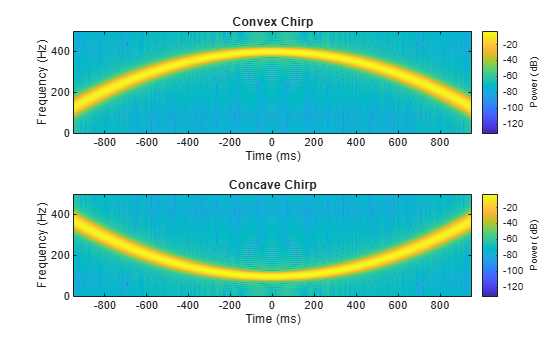Another function generator is the `vco` (voltage-controlled oscillator), which generates a signal oscillating at a frequency determined by the input vector. The input vector can be a triangle, a rectangle, or a sinusoid, among other possibilities.

Generate 2 seconds of a signal sampled at 10 kHz whose instantaneous frequency is a triangle. Repeat the computation for a rectangle.

```fs = 10000; t = 0:1/fs:2; x1 = vco(sawtooth(2*pi*t,0.75),[0.1 0.4]*fs,fs); x2 = vco(square(2*pi*t),[0.1 0.4]*fs,fs);```

Plot the spectrograms of the generated signals.

```figure subplot(2,1,1) spectrogram(x1,kaiser(256,5),220,512,fs,'yaxis') title('VCO Triangle') subplot(2,1,2) spectrogram(x2,256,255,256,fs,'yaxis') title('VCO Rectangle')```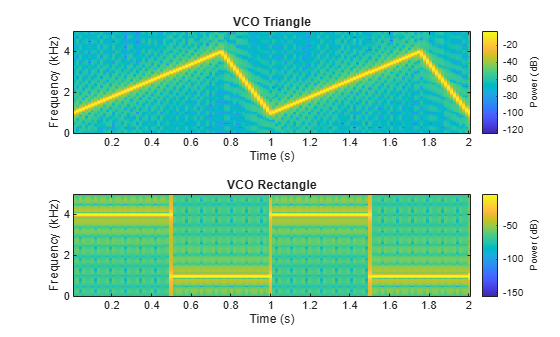### Pulse Trains

To generate pulse trains, you can use the `pulstran` function.

Construct a train of 2 GHz rectangular pulses sampled at a rate of 100 GHz at a spacing of 7.5 ns.

```fs = 100E9; % sample freq D = [2.5 10 17.5]' * 1e-9; % pulse delay times t = 0 : 1/fs : 2500/fs; % signal evaluation time w = 1e-9; % width of each pulse yp = pulstran(t,D,@rectpuls,w);```

Generate a periodic Gaussian pulse signal at 10 kHz with 50% bandwidth. The pulse repetition frequency is 1 kHz, the sample rate is 50 kHz, and the pulse train length is 10 milliseconds. The repetition amplitude should attenuate by 0.8 each time. Uses a function handle to specify the generator function.

```T = 0 : 1/50e3 : 10e-3; D = [0 : 1/1e3 : 10e-3 ; 0.8.^(0:10)]'; Y = pulstran(T,D,@gauspuls,10E3,.5); figure subplot(2,1,1) plot(t*1e9,yp); axis([0 25 -0.2 1.2]) xlabel('Time (ns)') ylabel('Amplitude') title('Rectangular Train') subplot(2,1,2) plot(T*1e3,Y) xlabel('Time (ms)') ylabel('Amplitude') title('Gaussian Pulse Train')```